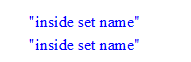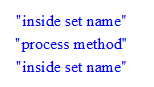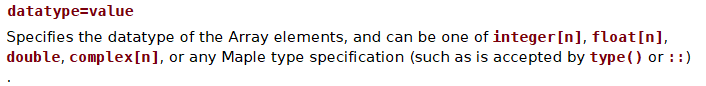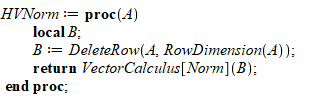## Evaluate the differentials...

Hi everyone:

How can I calculate these differentials? (a, b and R are constants).tnx...

I read the article "ONEOptimal: A Maple Package for Generating One-Dimensional Optimal System of Finite Dimensional Lie Algebra", and also searched out in Maplesoft website, but couldn't found. Does anyone have the package?

## what are the rules for naming object methods?...

As I am playing with Maple object to understand it better, I found strange thing.

I have simple object with 2 methods. Both are exported so they could be called from outside.

One method is called set_name and this one is called OK (I have print inside to print it is called). The next exported method is called process and when calling this method, the print never shows up. Which means it is not called. Using the same exact call.

When changing the name from process to something else, say processX now the print show, meaning the method is now called.

Is there restriction on what one can call their object method? And why would there be ?

Here is an example

restart;
module car_class()
option object;
local name::string:="";

export process::static := proc(o::car_class)
print("process method");
end proc:

export set_name::static := proc(o::car_class,_name::string)
print("inside set name");
o:-name := _name:
end proc:
end module:

my_car:=Object(car_class):  #make object

set_name(my_car,"toyota"):
process(my_car):
set_name(my_car,"toyota"):



On the screen, it only showsNow I changed it to

restart;
module car_class()
option object;
local name::string:="";

export processX::static := proc(o::car_class)
print("process method");
end proc:

export set_name::static := proc(o::car_class,_name::string)
print("inside set name");
o:-name := _name:
end proc:
end module:

my_car:=Object(car_class):
set_name(my_car,"toyota"):
processX(my_car):
set_name(my_car,"toyota"):


And now on the screen it shows OKIs there a way a way to be able to freely choose what method names to give to the object method? clearly the name "process"  is something special for Maple here for some reason.

When I do ?process, I see that Maple has some commands that have this name. But this is a name of a method inside Object class, so it should not have anything to do with any Maple own command.

This was never an issue with module() for an example.  If one has to worry about what name to give to their methods for an object, then this will be very awkward. For example, what if one calls their method FOO and future version of Maple introduce a new command called FOO now the code will no longer work.

## how to store Objects in an Array?...

I wanted to create an Array() to store only objects of specific type in it.

Array() supports the datatype=value  telling it the type of elements to store. But when I try to create an Array() using datatype of the class, it fails. I must be doing something wrong. Here is an example

restart;
module solution_class()
option object;
local sol::anything;
end module;
sol:=Object(solution_class); #create an object


Now the following works:

A:=Array();
A(1):=sol;


But I wanted to do

A:=Array(datatype=solution_class);
A(1) := sol;

Error, unable to store '0' when datatype=module solution_class () local sol::anything; option object; end module

Help saysAnd this is the case here, because type(sol,solution_class)  gives true

So 'solution_class' is valid type name.

Where is my error?

## How to plot a figure for different values of M?...

Dear maple users,

Greetings.

Q1.How to plot a figure for different values of M?

like M=1,2,3,4:

Code:Mplot.mw

Q2. How to assign all the expressions are "remember table assignment"## Value of the Expression...

Hi:

what is the value of this expression(eq) in Maple:

eq:=(x+I*y)^2-4*exp(I*x*y)

tnx...

## Error importing a big Excel data file xlsx...

Hello,

I try import a big Excel file with the first row as column labels for performing statistical etc.

I use this command :

data := Import(FileTools:-JoinPath(["d:", "directory", "subset2.xlsx"], platform = "windows"))

i need a dataframe for performing statistical with column label as first row, but i have this error :

Error, (in Import) invalid input: MatrixToDataFrame uses a 3rd argument, columns, which is missing

I can not understand what is this error...so, what i can do ?

Thanks, regards

## F:=VectorField(⟨y,-x,0⟩); Error, unknown operator...

Trying to use Curl tutorial when doing vector calculus

when I enter F:=VectorField(⟨y,-x,0⟩); it tells me unknown operator.

Regards

with(VectorCalculus);
SetCoordinates('cartesian'[x, y, z]);
cartesian[x, y, z]

F:=VectorField(⟨y,-x,0⟩);

Error, unknown operator
Typesetting:-mambiguous(FAssignVectorFieldApplyFunction(acirc

Typesetting:-mambiguous(?,

Typesetting:-merror("unknown operator"))umlycommauminus0xcomma0

acirc?copy))

## Situational VectorCalculus[Norm] argument passing ...

I am having problems with VectorCalculus[Norm] with argument passing.

In certain cases I get the error message:

"Error, (in VectorCalculus:-GetCoordinates) the first argument, when present, must be a Vector"

In all cases, the first argument IS a vector.

Here is the case I need to succeed:

VectorCalculus[Norm](DeleteRow(Vector[column](5, [x, y, z, w, 1]), RowDimension(Vector[column](5, [x, y, z, w, 1]))))

or more completely:here is the test Document with several cases of the problem explored:

Failure_on_Calculus_Verctor_Norm.mw

Background.  Calculus Vector Norm doesn't know about Homogenous systems so it inculdes an unwanted term in its norm.  Trimming off the last row of the vector fixes this problem.

(Note: as I am new, so if there is an existing cononical notation that will take care of that without further ado, then I could leave CalculusVector[Norm] to its own devices and move on.

Also, if I knew how to peek at the underside of such gotchas, I might have been able to sort it out myself.  Any leads in this direction would be taken to heart.  Thanks)

## how to explain the output of the function Automor...

I want to find AutomorphismGroup  of star S4 (ie, K1,3).

with(GraphTheory):
with(GroupTheory):
with(SpecialGraphs):
S4:=CompleteGraph(1,3);
G1 := AutomorphismGroup(S4); # out is GroupTheory:-PermutationGroup({module() ... end module,module() ... end module},degree=4)

thehas six automorphisms: (1, 2, 3), (1, 3, 2), (2, 1, 3), (2, 3, 1), (3, 1, 2,), (3, 2, 1):For the output of maple ,I cannot understand.

Maybe I don’t understand the group enough. May somebody explain in detail,  Thanks!

## How much is OO used in Maple? ...

There is a whole chapter in programming guide on OO in Maple.

How much is OO actually used by Maple programmers? Is OO used much internally at Maplesoft itself in implementing internal code? I do not see questions about OO in Maple in the forum. May be it has not taken off?

Any one knows of packages in Maple written in OO style, using the Object module? I think OO was added in Maple 16, which is 2012?

p.s. I myself like OO programming. Done alot of it in Java. I think it can be useful for large applications.

## How I can creat code to find coefficients of produ...

Hi I have product of function Q along with its derivative.

Q'j(x)*Qi(x) for j=0,1 and i=0,...n.

how can i make loop  to collect all coefficeints for this function for j=0,1 and i=0,1,..n.

i hope some one will help me.

Thanks

## motion of the conical pendulum...

Hello, i'm a newbie in maple. I need to simulate the movement of the conical pendulum.

The equations of motionandI include a link to a description of the conical pendulum.http://olewitthansen.dk/Physics/Conical_pendulum.pdf

## RMS of a function...

Hi,

How can I find the RMS of a function like x(t) in maple? the help is not clear

x(t) := -3.703703704*10^(-7)*(0.000111668023*cos(1000/33*sqrt(1122)*t) - 0.0001214712007*sin(1000/33*sqrt(1122)*t) - 0.0002325581396*sqrt(561)*sqrt(2)*(-0.0004467462845*sqrt(1122)*sin(1000/33*sqrt(1122)*t) + 0.0004467462845*sqrt(1122)*cos(1000/33*sqrt(1122)*t)))/((2.074226433*10^14*cos(1000/33*sqrt(1122)*t) + 2.074226433*10^14*sin(1000/33*sqrt(1122)*t))*(4.895037587*10^(-11) + 0.01685634229*(0.00001474262739*cos(1000/33*sqrt(1122)*t) + 0.00001474262739*sin(1000/33*sqrt(1122)*t))^2)^2);

Thanks,

Baharm31

## Are subscripts messing up my derivative?...

Sorry, I have not used Maple for a long time...

I would like to know the derivative of Jy w.r.t. py1 in the following. I expect the answer to be 2 Wy6 py1-2 Wy6 py10 but I get 0. Am I not using the subscripts properly that Maple is not understanding me?(1)(2)(3)